# Spatial autocorrelation (modelling)

Jérôme Guélat, Swiss Ornithological Institute (2013)

### Introduction

In the previous tutorial we have seen how spatial autocorrelation can be detected in a dataset, and especially in the residuals of a linear model. Residual spatial autocorrelation is a typical case of pseudoreplication which will lead to an overestimation of the precision of the results (eg. the standard errors and therefore also the confidence intervals, will be too small). This is due to the fact that autocorrelated measurements only artificially increase sample size without contributing independent information. Hence the real number of degrees of freedom is lower than the one used by the model.

Subsampling is the first solution to solve the autocorrelation problem, and was regularly used until some years ago. Basically you remove the points which are too close from one another and then you fit the model. If you can still detect residual spatial autocorrelation, then you need to remove more points. This solution is far from optimal and should not be used. You're losing a lot of information, and it is always a pity to throw away data when you've spent some many days in the field to acquire it.

A better solution consists of using statistical methods which allow us to account for (or even remove) spatial autocorrelation. All the methods I'm going to present assume spatial stationarity, which means that spatial autocorrelation and the effects of other covariates are expected to be constant across the region. This assumption may sometimes be problematic. Dormann et al. provides the following example: if the main cause of spatial autocorrelation is dispersal, stationarity will be violated if animals move to different habitats where movements are more or less restricted. Unfortunately, very few methods are available to account for non-stationarity.

Statisticians have been interested in spatial autocorrelation for quite a long time now, and they created a lot of different methods to account for it. In this tutorial I will present only a few of them that are useful for species distribution models (either because I like them and/or because they are easy to implement). In the first part I will ignore the problem caused by imperfect detection. In the second part I will show models which account for imperfect detection and spatial autocorrelation.

### Data generation

library(raster)

## Loading required package: sp

## Warning: package 'sp' was built under R version 3.0.2

library(gstat)
library(lattice)

## Warning: package 'lattice' was built under R version 3.0.2

library(nlme)

## Warning: package 'nlme' was built under R version 3.0.2

##
## Attaching package: 'nlme'
##
## The following object is masked from 'package:raster':
##
##     getData

set.seed(100)

# Define function to draw random samples from a multivariate normal
# distribution
rmvn <- function(n, mu = 0, V = matrix(1)) {
p <- length(mu)
if (any(is.na(match(dim(V), p))))
stop("Dimension problem!")
D <- chol(V)
t(matrix(rnorm(n * p), ncol = p) %*% D + rep(mu, rep(n, p)))
}

# Set up a square lattice region
simgrid <- expand.grid(1:50, 1:50)
n <- nrow(simgrid)

# Set up distance matrix
distance <- as.matrix(dist(simgrid))
# Generate random variable
delta <- 0.05
X <- rmvn(1, rep(0, n), exp(-delta * distance))

# Visualize results
Xraster <- rasterFromXYZ(cbind(simgrid[, 1:2] - 0.5, X))
par(mfrow = c(1, 2))
plot(1:100, exp(-delta * 1:100), type = "l", xlab = "Distance", ylab = "Correlation")
plot(Xraster)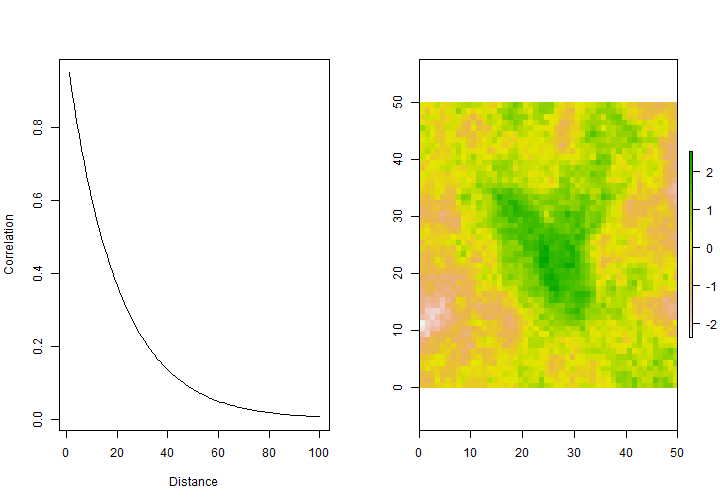Now let's import some environmental data from Switzerland. We first import the data as a text file, then convert the elevation data to a raster object. After that, we crop the layer to keep only a small part of the dataset. For convenience reasons we also align it with the simulated autocorrelated dataset, and the values are standardized.

# Import data
str(ch)

## 'data.frame':    42275 obs. of  5 variables:
##  $x : int 485500 485500 486500 486500 486500 486500 486500 486500 486500 486500 ... ##$ y        : int  109500 110500 109500 110500 111500 112500 116500 117500 118500 119500 ...
##  $elevation: int 340 340 380 390 357 357 500 472 462 472 ... ##$ forest   : int  6 11 4 72 9 5 0 43 6 0 ...
##  $water : int 0 1 0 3 7 5 0 0 0 0 ...  ch$x <- ch$x/1000 ch$y <- ch$y/1000 # Convert to raster object elev <- rasterFromXYZ(ch[, c("x", "y", "elevation")]) ext <- extent(c(600, 650, 160, 210)) # Crop and shift the raster elev <- crop(elev, ext) elev <- shift(elev, x = -600, y = -160) # Standardize the elevation values elev <- scale(elev) # Convert the raster object to a SpatialPixelsDataFrame for later use elevpx <- as(elev, "SpatialPixelsDataFrame") plot(elev, main = "Elevation")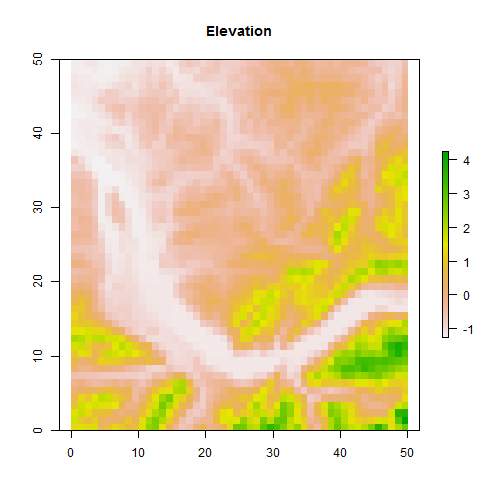We will now simulate the abundance distribution of some animal species. We choose a quadratic effect of elevation and compute the expected abundance values. We also compute a second set of expected values by including an effect of another spatially autocorrelated covariate. # Define coefficients beta0 <- 1 beta1 <- 3 beta2 <- -2 # Abundance as a quadratic function of elevation lambda1 <- exp(beta0 + beta1 * values(elev) + beta2 * values(elev)^2) # Abundance as a quadratic function of elevation (+ the other spatially # autocorrelated covariate) lambda2 <- exp(beta0 + beta1 * values(elev) + beta2 * values(elev)^2 + values(Xraster)) # Plot the results par(mfrow = c(1, 2)) plot(values(elev), lambda1, cex = 0.5, main = expression(lambda == f(elevation))) plot(values(elev), lambda2, cex = 0.5, main = expression(lambda == f(elevation, X)))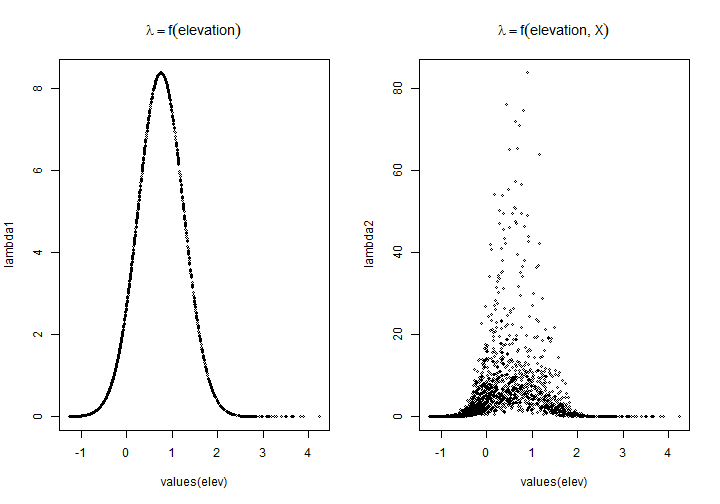The points of the second response curve are much more dispersed around the quadratic effect of elevation. This is of course expected because the expected abundances are not only modelled as a function of elevation, but also of our simulated covariate. We can now compute the actual counts using the second set of expected values. This is what we would observe if we would visit every pixel and count all individuals from that species (and assuming we don't miss any individual, ie. detection probability = 1). # Determine the actual counts counts <- rpois(n, lambda2) counts <- rasterFromXYZ(cbind(coordinates(elev), counts)) plot(counts, main = "Abundance distribution")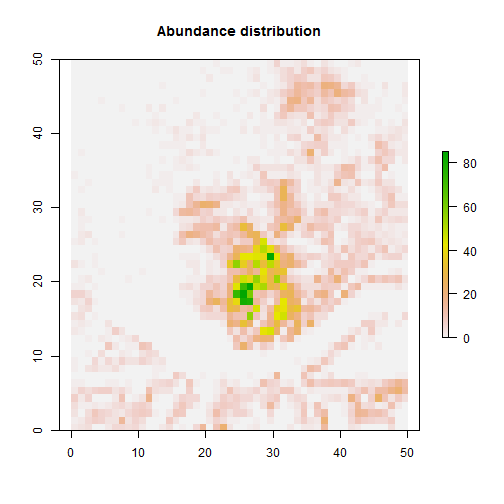Let's imagine we are able to visit 200 sites in our study area and count the birds which are present at these sites. We randomly choose 200 pixels and extract the related abundance values. We will then use this dataset for our subsequent analyses. coords <- coordinates(counts) fulldata <- data.frame(coords, elevation = extract(elev, coords), Xvar = extract(Xraster, coords), counts = extract(counts, coords)) sites <- sample(1:n, 200) sampledata <- fulldata[sites, ] head(sampledata)  ## x y elevation Xvar counts ## 1506 5.5 19.5 -1.0460 -1.15275 0 ## 1645 44.5 17.5 -1.1372 0.49944 0 ## 2255 4.5 4.5 1.8993 0.06859 0 ## 304 3.5 43.5 -1.0600 -0.28208 0 ## 1438 37.5 21.5 0.9449 0.16388 2 ## 2267 16.5 4.5 1.1811 -0.03190 6  plot(counts, main = "Abundance distribution with sampling sites") points(sampledata[, 1:2], pch = 16)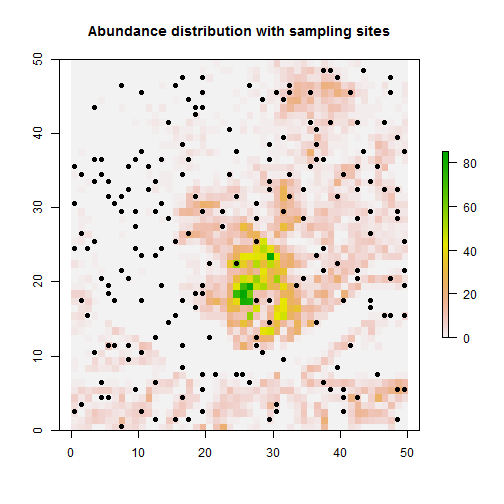### Analysis using GLS As I said in the introduction, the best solution in this case would be to find the missing covariate and to include it in the model. Unfortunately this is not always possible and we have to find another solution. One possibility is to model this residual spatial autocorrelation and to include this model in our linear model of abundance. To account for spatial autocorrelation, we will use a procedure called GLS (generalised least-squares) available in the package nlme. Actually, the GLS directly models the spatial covariance structure in the variance-covariance matrix using parametric functions. But first we'll ignore spatial autocorrelation and re-fit the model we had in the introduction, this time using the gls function (instead of lm). The results will be the same, but we will need this model later when doing model comparisons using AIC (i.e. we can't compare the AICs from the model fit using lm with that fit using gls). The nlme package also offers a variogram function. m2 <- gls(log1p(counts) ~ elevation + I(elevation^2), data = sampledata) vario2 <- Variogram(m2, form = ~x + y, resType = "pearson") plot(vario2, smooth = TRUE, ylim = c(0, 1.2))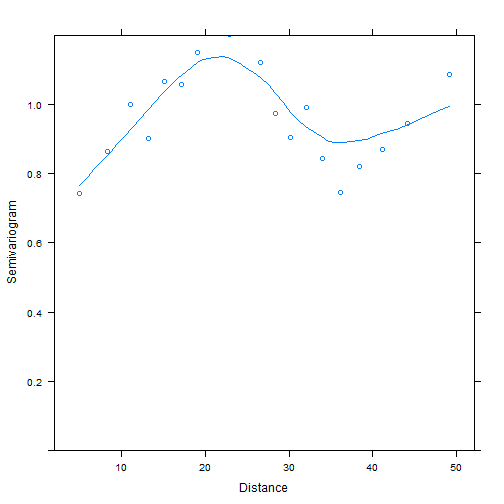On the variogram we see that the semi-variance is clearly increasing with distance. We have a confirmation that spatial autocorrelation is present in our residuals. Now let's fit our model with a spatial correlation structure. This is done within the gls function using the correlation argument. We fit our model using different correlation structures, and we then use AIC to choose the best model (we also compare the initial model without correlation). Note that, as with the Variogram function, we need two columns in our dataframe containing the coordinates of our sites. The nugget argument allows us to choose wether we want a nugget effect (intercept) or not. m3 <- gls(log1p(counts) ~ elevation + I(elevation^2), correlation = corExp(form = ~x + y, nugget = TRUE), data = sampledata) m4 <- gls(log1p(counts) ~ elevation + I(elevation^2), correlation = corGaus(form = ~x + y, nugget = TRUE), data = sampledata) m5 <- gls(log1p(counts) ~ elevation + I(elevation^2), correlation = corSpher(form = ~x + y, nugget = TRUE), data = sampledata) m6 <- gls(log1p(counts) ~ elevation + I(elevation^2), correlation = corLin(form = ~x + y, nugget = TRUE), data = sampledata)  ## Error: false convergence (8)  m7 <- gls(log1p(counts) ~ elevation + I(elevation^2), correlation = corRatio(form = ~x + y, nugget = TRUE), data = sampledata) AIC(m2, m3, m4, m5, m6, m7)  ## Error: object 'm6' not found  In our case, the exponential correlation structure seems to be the most appropriate one (the corRation structure is a close runner-up). This is not really a big surprise since we've been using the exponential model to generate our missing covariate. Note that, depending on your data and model, some correlation structures are more difficult to fit and you may thus get an error message. We can also plot the fitted variogram to check if the fit is OK. vario3 <- Variogram(m3, form = ~x + y, resType = "pearson") plot(vario3, smooth = FALSE, ylim = c(0, 1.2))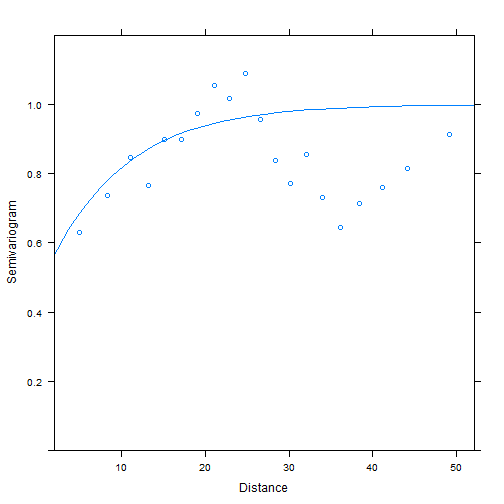It looks pretty good. As a last check, we plot a sample variogram of the normalized residuals. These residuals are standardized residuals pre-multiplied by the inverse square-root factor of the estimated error correlation matrix. Hence we shouldn't see any trend in this new variogram if the residual spatial autocorrelation was properly accounted for. vario4 <- Variogram(m3, form = ~x + y, resType = "normalized", maxDist = 30) plot(vario4, smooth = FALSE, ylim = c(0, 1.2))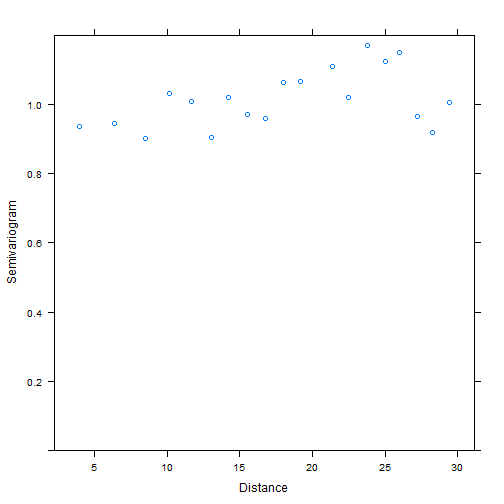We see more or less a horizontal band of points, therefore we can conclude that our model fits our data in an appropriate way and we can start to make inferences based on these results. Note that accounting for spatial autocorrelation using the gls function allows us to get unbiased parameter and uncertainty estimates. However, the modelled correlation structure is not directly used when making predictions. If we want to use the extra-information provided by spatial autocorrelation to make better predictions, we need more complex models… resp <- expm1(predict(m3, newdata = data.frame(elevation = values(elev), x = coordinates(elev)[, 1], y = coordinates(elev)))) resp <- rasterFromXYZ(cbind(coordinates(elev), resp)) plot(resp, main = "Predicted abundances")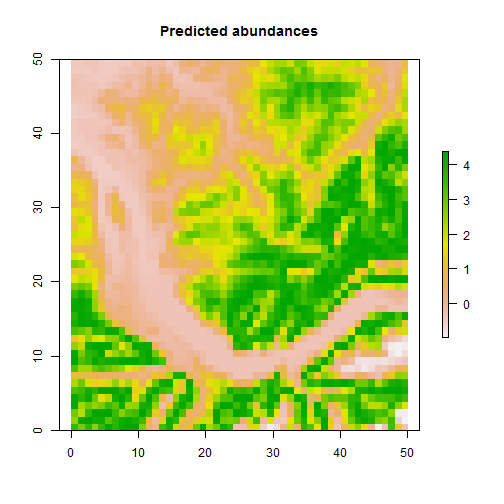### Analysis using ordinary kriging The term kriging brings together a whole family of models which, based on a variogram, provide a way to estimate a spatial random field at unobserved locations. These techniques are also known as Gaussian process regression or best linear unbiased prediction. The basic idea is the following: the value of interest at an unknown point is computed as a weighted average of the sampled neighbours. The weights are defined by the variogram model. Ordinary kriging (and universal kriging, see next section) assumes that the data comes from a multivariate normal distribution. That's why we use a log transformation in this example. # Convert dataset to SpatialPointsDataFrame datsp <- sampledata coordinates(datsp) <- c("x", "y") # Compute the sample variogram of our data (without covariate) vario <- variogram(log1p(counts) ~ 1, datsp) plot(vario)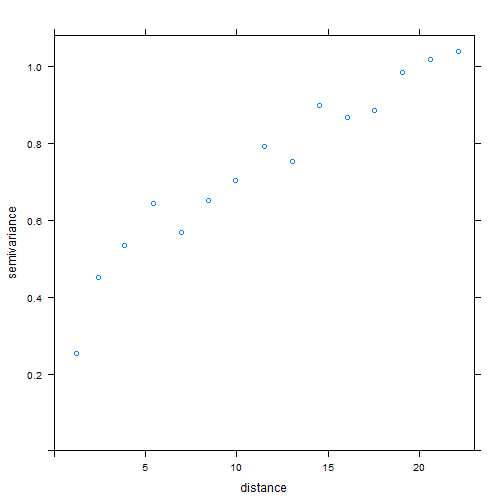Ordinary kriging is the simplest kriging method, we only need to know the relationship between similarity and distance (we don't need any covariate). This relationship is computed from the sample variogram. To get predictions we need to fit a theoretical variogram model to our sample variogram (we did something similar with the gls function). We actually fit a non-linear regression to our variogram using the fit.variogram function of the gstat package. The vgm function allows us to specify the theoretical model (e.g. exponential, gaussian, spherical, etc.) and also to give initial values for the variogram parameters. # Fit a theoretical variogram model to our sample variogram counts.ivgm <- vgm(model = "Exp", nugget = 0, range = 15, psill = 1) counts.vgm <- fit.variogram(vario, model = counts.ivgm) counts.vgm  ## model psill range ## 1 Nug 0.1659 0.000 ## 2 Exp 0.8025 7.286  plot(vario, counts.vgm, pch = "+", main = "log1p(counts)", cex = 2)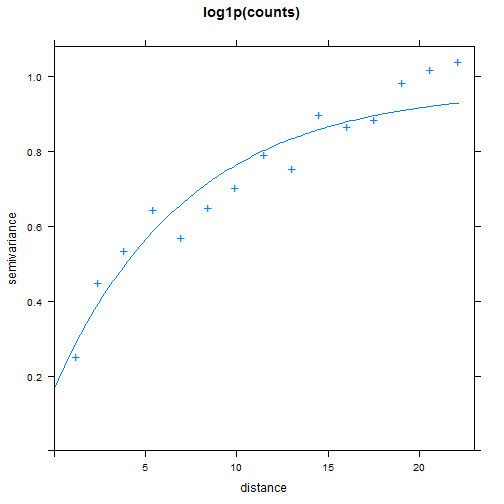Once we have modelled the variogram, we can use it to make predictions. The first argument of the krige function is the formula, the “~1” part indicates that we want to use ordinary kriging. The second argument is a SpatialPointsDataFrame containing the locations of the sampled sites with the corresponding values of the response variable. The third argument is Spatial object (in this case a SpatialPixelsDataFrame) containing the coordinates of the sites for which we want to have predictions. The last argument is the fitted variogram model. The krige function will return a SpatialPixelsDataFrame with 2 columns. The first one contains the predictions and the second one contains the prediction variances. Because we used a log transformation to fit the model, we also need to back-transform the predictions. # Ordinary kriging counts.ok <- krige(log1p(counts) ~ 1, locations = datsp, newdata = elevpx, model = counts.vgm)  ## [using ordinary kriging]  preds <- stack(counts.ok) plot(preds, main = c("Ordinary kriging predictions", "Prediction variance"))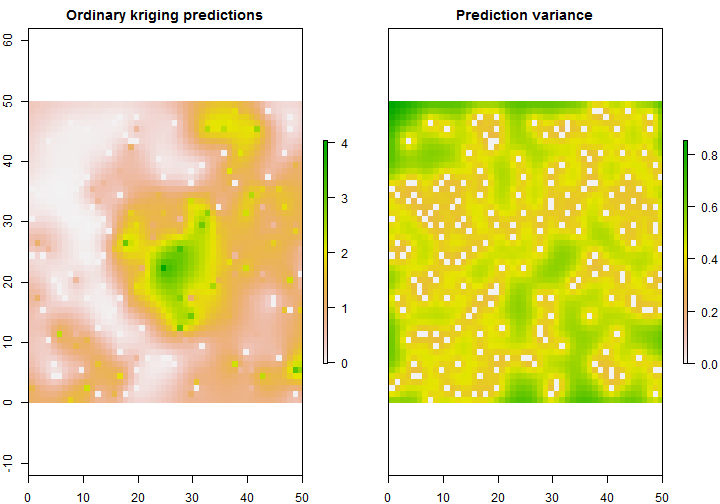# Back-transform the predictions plot(exp(preds[]) - 1, main = "Predictions (back-transformed")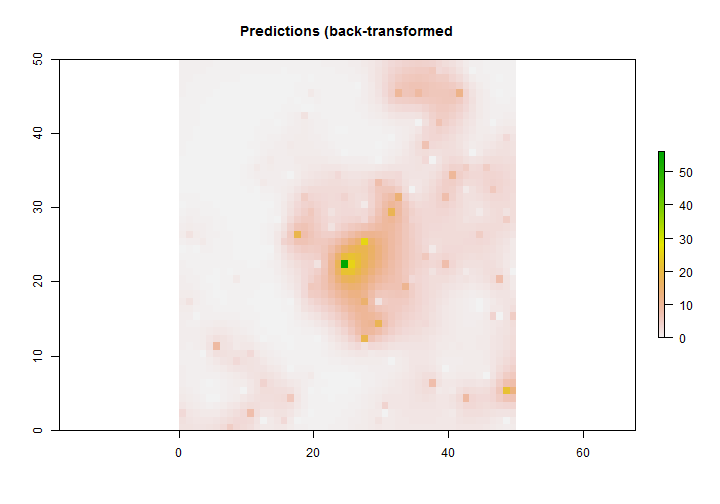Because we don't use any covariate, the predictions are usually strongly smoothed and most of the time not very accurate. Therefore this method doesn't work well if the distribution of a species is mostly determined by covariates with sharp boundaries. ### Analysis using universal kriging (regression kriging) Adding covariates is of course a logical improvement to ordinary kriging. This is called universal kriging (or regression kriging, or kriging with external drift) and it a really nice technique to combine information coming from the covariates and information coming from the residual spatial autocorrelation. Universal kriging also assumes that the data comes from a multivariate normal distribution (but see below). Actually the model fitting is mathematically identical to the GLS procedure with covariates, but the prediction part is different. # Fit a simple linear model m <- lm(log1p(counts) ~ elevation + I(elevation^2), data = datsp) # Compute the sample variogram of the residuals: residual spatial # autocorrelation vario.rs <- variogram(residuals(m) ~ 1, datsp) # Fit a theoretical variogram model to the residuals, and give initial # values counts.rivgm <- vgm(model = "Exp", nugget = 0, range = 10, psill = 0.5) counts.rvgm <- fit.variogram(vario.rs, model = counts.rivgm) counts.rvgm  ## model psill range ## 1 Nug 0.1783 0.000 ## 2 Exp 0.2858 7.735  plot(vario.rs, counts.rvgm, pc = "+", main = "Residuals", cex = 2)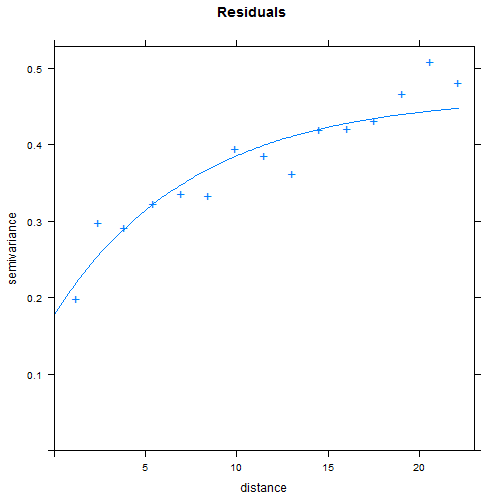We now have a theoretical model describing the residual spatial autocorrelation. We can again use the krige function, but this time with covariates in the formula. When using universal kriging, the third argument must not only contain the coordinates of the predicted sites, but also the value of the covariates for these sites. As with ordinary kriging, the krige function will return a SpatialPixelsDataFrame with 2 columns. The first one contains the predictions and the second one contains the prediction variances. Because we used a log transformation to fit the model, we also need to back-transform the predictions. # regression-kriging counts.uk <- krige(log1p(counts) ~ elevation + I(elevation^2), datsp, elevpx, counts.rvgm)  ## [using universal kriging]  preds <- stack(counts.uk) plot(preds, main = c("Universal kriging predictions", "Prediction variance"))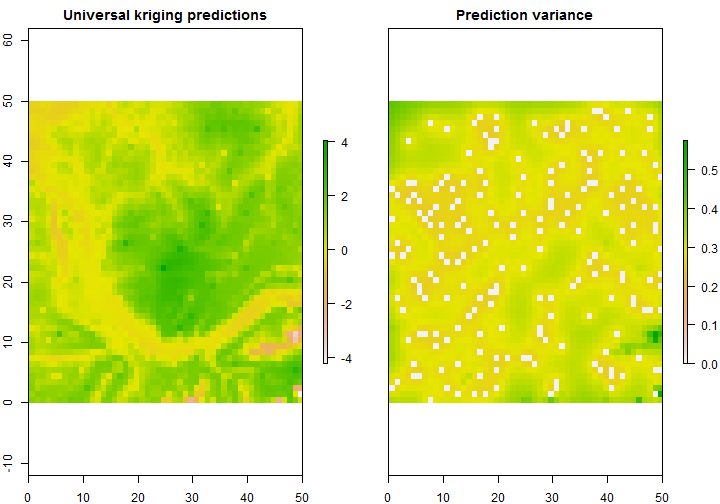plot(exp(preds[]) - 1, main = "Predictions (back-transformed")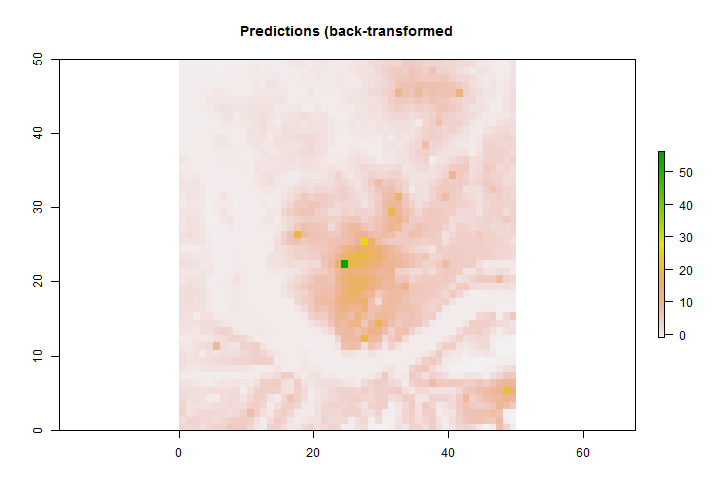Universal kriging is mathematically equivalent to the following 2-step procedure: first fit a linear a model to your data using covariates, then perform ordinary kriging on the residuals and add the kriged residuals to the predictions of your linear model. The new predictions you will get are the same as the ones provided by the universal kriging procedure. This way of computing universal kriging predictions is usually called regression kriging. We immediately see one of the main advantages of this alternative parameterisation : it is possible to fit a GLM (generalised linear model) to our count data using, for example, a Poisson distribution; we can then interpolate the GLM residuals using ordinary kriging and add them to our predictions. Of course we should first add the kriged residuals to the predictions on the link scale, and then back-transform the results. Unfortunately, it is much more complicated to get the corresponding standard errors using this method. The variogram fitting is sometimes a difficult task, especially if we are not familiar with the different theoretical models. The automap package provides the very useful autokrige function. It will automatically fit the most appropriate variogram model (with the smallest residual sum of squares) and compute predictions using kriging. Most of the time this function will fit more flexible variogram models (Matern models). # Automatic variogram fitting library(automap) counts.uk <- autoKrige(log1p(counts) ~ elevation + I(elevation^2), datsp, elevpx)  ## [using universal kriging]  ## Warning: NaNs produced  plot(counts.uk)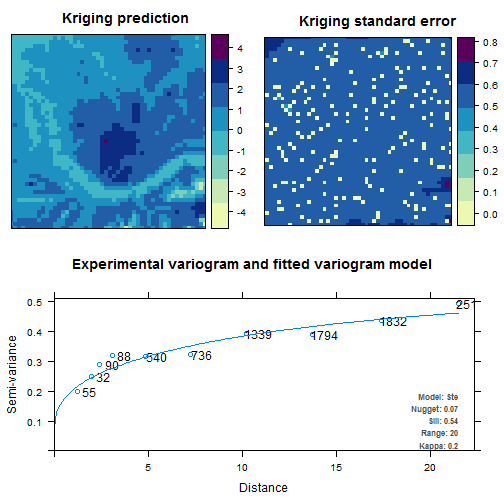The object returned by the autoKrige is a little more complex than the one returned by the standard krige function. It contains the kriging predictions and variance as a SpatialPixelsDataFrame, and the fitted variogram. In order to back-transform our predictions, we first need to extract the kriging predictions. kpreds <- counts.uk$krige_output
preds <- stack(kpreds)
plot(exp(preds[]) - 1, main = "Predictions (back-transformed")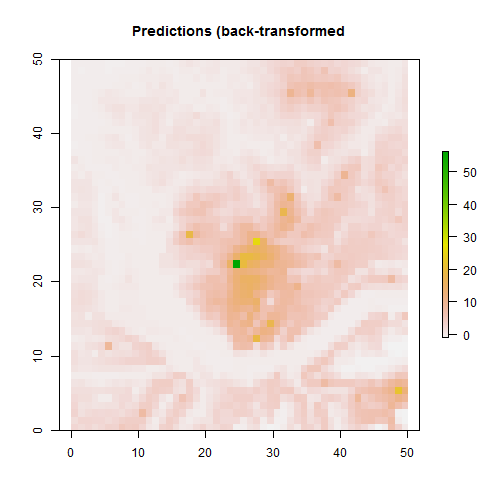### Analysis using Conditional Autoregressive (CAR) models

Kriging is modelling spatial autocorrelation as a continuous process. This is of course more realistic for most ecological analyses but it can be very computationally expensive, especially in a bayesian context (see the spatial.exp function in WinBUGS). One solution is to model spatial autocorrelation as a discrete process and to assume that only the nearest neighbours are responsible for the residual spatial autocorrelation. This is exactly what Conditional Autoregressive (CAR) models are doing (we will only use the intrinsic CAR (ICAR) model).

First we need to prepare the data. Because we are using WinBUGS to fit the model, we can use NAs for the response variable in order to get predictions (i.e. all the unsurveyed sites will get a NA for the counts). Because spatial autocorrelation is modelled as a discrete process we don't need to use the distances between sites in our model, but we still need to define the direct neighbours for each site. This is done with the dnearneigh function from the spdep package. The first argument is a matrix containing the coordinates of the sites (including the ones for which we want predictions). The second argument is the minimal distance needed between sites to consider them as neighbours, and the third argument is the maximal distance. Our sites are on a grid with a resolution of 1, thus by using a minimal distance of 0 and a maximal distance of 1.5, each site will get 8 neighbours (the 8 pixels directly touching the site), except those on the border of the study area.

library(spdep)

## Warning: package 'spdep' was built under R version 3.0.2

## Loading required package: Matrix

## Warning: package 'Matrix' was built under R version 3.0.2

library(R2WinBUGS)

## Loading required package: coda
##
## Attaching package: 'boot'
##
## The following object is masked from 'package:lattice':
##
##     melanoma


# Define the sampled sites and add a missing response for the other ones
wbdata <- fulldata
wbdata[-sites, "counts"] <- NA

# Compute the neighbourhood data (2nd order: 8 neighbours)
nb <- dnearneigh(as.matrix(wbdata[, 1:2]), 0, 1.5)
table(card(nb))

##
##    3    5    8
##    4  192 2304

# Convert the neighbourhood object in an object usable by WinBUGS
winnb <- nb2WB(nb)


Now we have to define our model in the BUGS language. We start by defining a standard Poisson regression (because we are modelling counts) and we add a special type of random effect ($$\rho_i$$) in the linear predictor. The value of this random effect will be different for each site, and it is defined by the neighbouring sites. Here's the formal definition:

$\rho_i|\rho_w \sim \mbox{Normal}(\frac{1}{n_w}\sum{\rho_w}, \frac{\tau^2}{n_w})$

Basically the value of the random effect for each site is drawn from a normal distribution. The mean of the normal distribution is equal to the average of the random effects of the neighbours, and the variance is a parameter of the model (\tau2) which is scaled by the number of neighbours. This variance parameter controls the amount of variation between the random site effects.

The Poisson GLM we are going to use in order to model counts is thus the following:

$C_i \sim \mbox{Poisson}(\lambda_i)$ $log(\lambda_i) = \beta_0 + \beta_1x_i + \beta_2x_i^2 + \rho_i$

In WinBUGS we can use the car.normal function to fit CAR models. In order to compute the random effects, you need to specify the neighbours as arguments of the function (you can also give specific weights for the neighbours).

Please note that the car.normal function used in the following model is only available in WinBUGS and in OpenBUGS (not in JAGS). It is actually possible to manually code the CAR model in JAGS because the CAR models are actually using a multivariate normal distribution with a special covariance matrix. However, this is quite complicated and those models take a very very long time to run.

# Specify model in BUGS language
sink("CAR.txt")
cat("
model {

# likelihood
for (i in 1:n) {
y[i] ~ dpois(lambda[i])
log(lambda[i]) <- rho[i] + beta1*x1[i] + beta2*x2[i] + beta0
}

# CAR prior distribution for spatial random effect:
rho[1:n] ~ car.normal(adj[], weights[], num[], tauSp)

# other priors
beta0 ~ dnorm(0, 0.01)
beta1 ~ dnorm(0, 0.01)
beta2 ~ dnorm(0, 0.01)
tauSp <- pow(sdSp, -2)
sdSp ~ dunif(0, 5)
}

", fill = TRUE)
sink()


We can now prepare the data for WinBUGS and set the different MCMC settings. It is important to specify initial values for the CAR random effects. Using 0 for all the sites (including the sites used for predictions) is usually a good choice.

# Bundle data
win.data <- list(n = n, y = wbdata$counts, x1 = wbdata$elevation, x2 = (wbdata$elevation)^2, num = winnb$num, adj = winnb$adj, weights = winnb$weights)
# Initial values
inits <- function() {
list(beta0 = runif(1, -3, 3), beta1 = runif(1, -3, 3), beta2 = runif(1,
-3, 3), rho = rep(0, n))
}
# Parameters monitored
params <- c("beta0", "beta1", "beta2", "tauSp", "lambda", "rho")

# MCMC settings
ni <- 5000
nt <- 5
nb <- 2000
nc <- 3


We can now run the model in WinBUGS:

# Call WinBUGS from R (for R2OpenBUGS, just remove the 'bugs.dir' argument)
out <- bugs(data = win.data, inits = inits, parameters.to.save = params, model.file = "CAR.txt",
n.chains = nc, n.thin = nt, n.iter = ni, n.burnin = nb, bugs.dir = "C:/Program Files (x86)/WinBUGS14/",
working.directory = getwd(), clearWD = TRUE)


We can have a look at the parameter estimates and we can also extract the predictions from the object returned by R2WinBUGS. To get a nice map, we can convert the predictions to a raster object using the rasterFromXYZ function. Of course it is also possible to visualize the values of the spatial random effects on map.

# Get the posterior means of the model parameters
means <- c(out$mean$beta0, out$mean$beta1, out$mean$beta2, out$mean$tauSp)
means

##   1.2266  2.7701 -1.8204  0.6308

# Store results in raster objects and plot them
rcar <- rasterFromXYZ(cbind(wbdata[, 1:2], out$mean$lambda))
rrho <- rasterFromXYZ(cbind(wbdata[, 1:2], out$mean$rho))

plot(stack(rcar, rrho))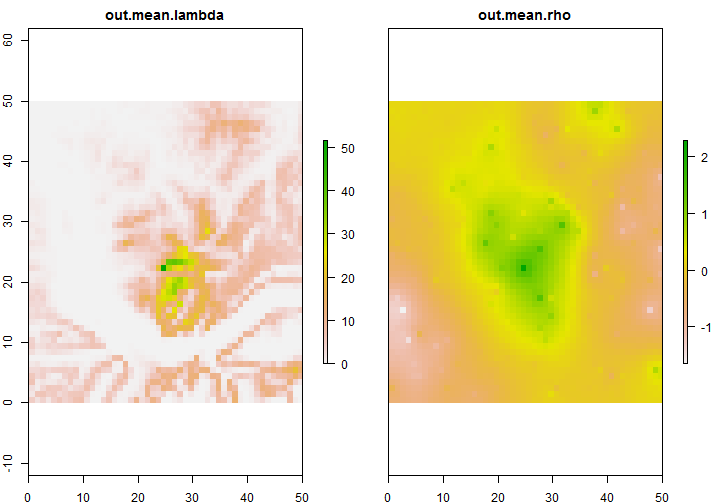The predictions look pretty good if we compare them with reality. If we have a closer look at the map of the spatial random effects, we can almost see the variable which was used to simulate the counts but wasn't included in the model.

Because we are using bayesian statistics, it is totally easy to get information about the uncertainty of the predictions. We should not forget that we have the full posterior distribution of the predictions for each site (we only plotted the mean of the posterior distributions on the previous map). The following code allows us to produce a map of the standard error (= the standard deviation of the posterior) associated to each prediction. We also produce maps of the bayesian 95% percent credible intervals.

lambdasd <- out$sd$lambda
rsd <- rasterFromXYZ(cbind(wbdata[, 1:2], lambdasd))

plot(rsd, main = "Standard errors")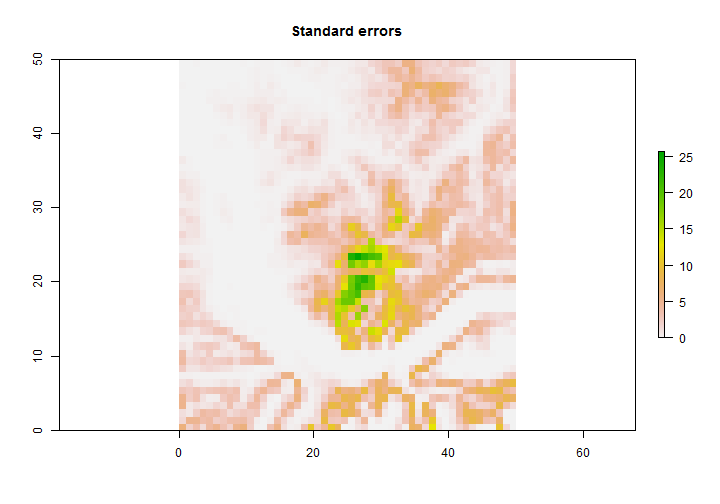lambdasims <- out$sims.list$lambda
lowerCI <- apply(lambdasims, 2, quantile, probs = 0.025)
upperCI <- apply(lambdasims, 2, quantile, probs = 0.975)

rlowerCI <- rasterFromXYZ(cbind(wbdata[, 1:2], lowerCI))
rupperCI <- rasterFromXYZ(cbind(wbdata[, 1:2], upperCI))

plot(stack(rlowerCI, rupperCI))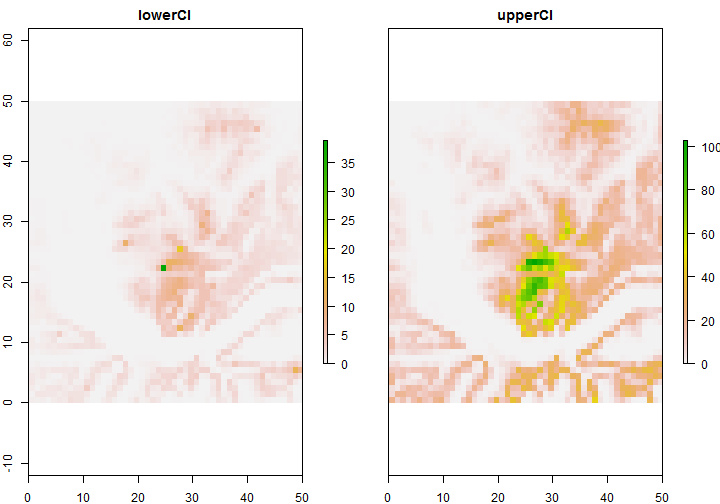CAR models are really useful to integrate spatial autocorrelation in your predictions. They are actually quite fast in comparison to other spatial models (e.g. bayesian kriging). Unfortunately you won't be able to fit them using WinBUGS or OpenBUGS on really big datasets because of lack of memory. This is due to the fact that these softwares were programmed using an old programming language and they are only compiled for 32 bits systems. Therefore you can have a huge amount of RAM on your computer but WinBUGS will only be able to use around 3 gb. One possible solution is to use another software called INLA (http://www.r-inla.org) to fit these models. However you won't be able to fit site-occupancy or N-mixture models in INLA.

### Analysis using N-mixture models with a CAR prior

Most of the time we will miss some individuals when we survey a site. This means that we don't know the real abundances but only some index of abundance. Using N-mixture models we can account for imperfect detection and get better estimates of the real abundances. If we suspect spatial autocorrelation might be a problem, it is of course possible to add a CAR random effect in our model. First let's simulate our observed counts: each site is surveyed three times, and the detection probability is 0.5.

# Simulate imperfect detection of the abundance
y <- matrix(nrow = n, ncol = 3)
# Detection probability
p <- 0.5
for (i in 1:3) {
y[, i] <- rbinom(n = n, size = fulldata$counts, prob = p) } # Have a look at the full dataset head(y)  ## [,1] [,2] [,3] ## [1,] 0 0 0 ## [2,] 0 0 0 ## [3,] 0 0 0 ## [4,] 0 0 0 ## [5,] 0 0 0 ## [6,] 0 0 0  # Use NAs for all unsurveyed sites ysample <- y ysample[-sites, ] <- NA # ysample must be a matrix otherwise WinBUGS will crash class(ysample)  ##  "matrix"  We can now write the model in the BUGS language. As you can see, this is only a mix of the standard N-mixture model and the previous CAR model. We ususally add a spatial random effect in the linear predictor defining the expected latent abundance, but it is of course possible to add another spatial random effect in the observation process. However you may have some problems of convergence and/or identifiability. We are going to use the following model: Model for occurrences: $N_i \sim \mbox{Poisson}(\lambda_i)$ $log(\lambda_i) = \beta_0 + \beta_1x_i + \beta_2x_i^2 + \rho_i$ $\rho_i|\rho_w \sim \mbox{Normal}(\frac{1}{n_w}\sum{\rho_w}, \frac{\tau^2}{n_w})$ Model for observations: $y_{ij} \sim \mbox{Binomial}(N_i, p)$ # Specify model in BUGS language sink("CAR_Nmix.txt") cat(" model { # likelihood for (i in 1:n) { N[i] ~ dpois(lambda[i]) log(lambda[i]) <- rho[i] + beta1*x1[i] + beta2*x2[i] + beta0 for (j in 1:nrep) { C[i,j] ~ dbin(p, N[i]) } } # CAR prior distribution for spatial random effects: rho[1:n] ~ car.normal(adj[], weights[], num[], tauSp) # other priors beta0 ~ dnorm(0, 0.01) beta1 ~ dnorm(0, 0.01) beta2 ~ dnorm(0, 0.01) tauSp <- pow(sdSp, -2) sdSp ~ dunif(0, 5) p ~ dunif(0, 1) } ", fill = TRUE) sink()  We can now prepare the data for WinBUGS and set the different MCMC settings. It is important to specify “good” initial values for this model otherwise you'll get a trap in WinBUGS # Bundle data win.data <- list(n = n, nrep = 3, C = ysample, x1 = wbdata$elevation, x2 = (wbdata$elevation)^2, num = winnb$num, adj = winnb$adj, weights = winnb$weights)
# Initial values
Nst <- apply(ysample, 1, max) + 1
Nst[is.na(Nst)] <- 1
inits <- function() {
list(beta0 = runif(1, -5, 5), beta1 = runif(1, -5, 5), beta2 = runif(1,
-5, 5), p = runif(1), N = Nst, rho = rep(0, n))
}

# Parameters monitored
params <- c("beta0", "beta1", "beta2", "tauSp", "lambda", "p")

# MCMC settings
ni <- 5000
nt <- 5
nb <- 2000
nc <- 3


We can now run the model in WinBUGS. Don't forget to add DIC = FALSE in the bugs command, otherwise the model won't run!

# Call WinBUGS from R (don't forget DIC = FALSE, otherwise you'll get a
# trap)
out <- bugs(data = win.data, inits = inits, parameters.to.save = params, model.file = "CAR_Nmix.txt",
n.chains = nc, n.thin = nt, n.iter = ni, n.burnin = nb, bugs.dir = "C:/Program Files (x86)/WinBUGS14/",
working.directory = getwd(), clearWD = TRUE, DIC = FALSE)


Once again, we can have a look at the parameter estimates and we can also extract the predictions from the object returned by R2WinBUGS. We also produce a map of the standard errors.

# Get the posterior means
means <- c(out$mean$beta0, out$mean$beta1, out$mean$beta2, out$mean$p, out$mean$tauSp)
means

##   1.1431  2.6883 -1.8105  0.5316  0.5879

# Store results in raster objects and plot them
rcar <- rasterFromXYZ(cbind(wbdata[, 1:2], out$mean$lambda))
rsd <- rasterFromXYZ(cbind(wbdata[, 1:2], out$sd$lambda))

plot(stack(rcar, rsd), main = c("Predictions", "Standard errors"))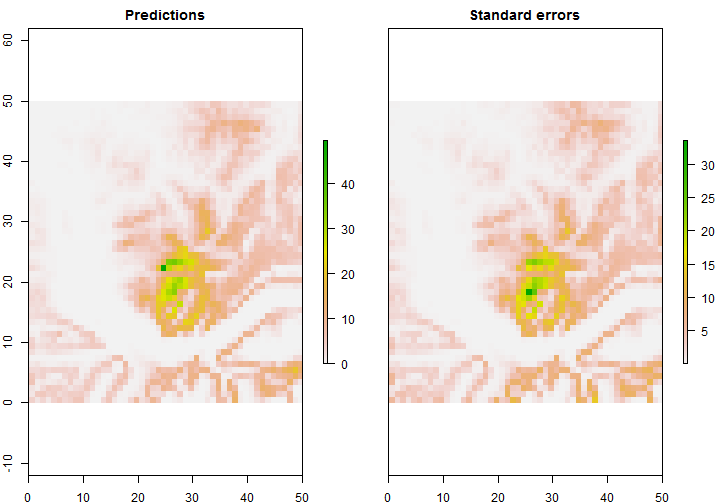We see that the N-mixture model was perfectly able to account for the imperfect detection since the predicted abundances are prettly close to the real ones (see the simulated map). Moreover the CAR random effect is also improving the predictions.

### Analysis using the hSDM package

The hSDM package allows us to fit site-occupancy models with a CAR random effect in the occurrence process. This package is using bayesian statistics to fit the model but it is amazingly fast thanks to a really efficient MCMC sampler coded in C.

First we need to prepare the data. In the previous examples, we were using abundance data but we'd like to work with occupancy now. That's why we modify our dataset by replacing all values bigger than 0 with 1. The hSDM package doesn't accept a two-dimensional array containing the detection histories for each site. Instead we have to use a vector containing the number of detections for each site. We also have to provide another vector containing the number of visits for each site.

If we want to do predictions, we can't use NAs as in WinBUGS. We have to use a 0 in the detection vector and of course another 0 in the “number of visits” vector.

library(hSDM)

## ##
## ## hSDM R package
## ## For hierarchical Bayesian species distribution models
## ##
##
##
## Attaching package: 'hSDM'
##
## The following object is masked from 'package:boot':
##
##     inv.logit, logit

# Transform count data into detection/non-detection data
yoccsample <- ysample
yoccsample[yoccsample > 0] <- 1
# Summarize the 3 surveys in 1 vector
presences <- apply(yoccsample, 1, sum)

# Create a new vector with the number of visits for each site
trials <- rep(3, n)
# The unsurveyed sites get a 0
trials[is.na(presences)] <- 0
# Replace the NAs with 0
presences[is.na(presences)] <- 0

# Create some site ID needed by hSDM
cells <- 1:n

# Create a dataframe containing the environmental variables
dat <- data.frame(elevation = fulldata$elevation, elevation2 = fulldata$elevation^2)

##   elevation elevation2
## 1    -1.196      1.430
## 2    -1.177      1.385
## 3    -1.126      1.267
## 4    -1.135      1.288
## 5    -1.196      1.430
## 6    -1.247      1.555


We can now fit the model using the hSDM.hierarchical.binomial function. The suitability argument contains the formula for the occurrence process. The observability argument contains another formula for the observation process. Because we want a constant detection probability for all the sites, we don't use any covariate to model detection probability in this example. We also have to specify the neighbours for each site. This is done with the same object which we used in WinBUGS. Because it is a bayesian analysis, we also need to specify a burnin, the number of iterations, the thinning rate and some initial values.

newCAR <- hSDM.hierarchical.binomial(presences = presences, trials = trials,
suitability = ~elevation + elevation2, cells = cells, n.neighbors = winnb$num, neighbors = winnb$adj, alteration = rep(0, n), observability = ~1, data = dat,
burnin = 5000, mcmc = 40000, thin = 4, beta.start = c(0, 0, 0), gamma.start = 0,
Vrho.start = 1, verbose = FALSE)

##
## Running the Gibbs sampler. It may be long, please keep cool :)


The object returned by the function is a list containing four elements. The first one contains the MCMC chains for each parameter, the second one contains the estimated values of the spatial random effect for each site (including the predicted ones), the third one contains the estimated occupancy values, and the fourth one contains the estimates detectability values.

The following code show how to have a look at the MCMC chains and how to extract the predictions to make nice maps. Because we have access to the MCMC chains, it would also be easy to produce uncertainty maps.

# Have a look at the returned object
str(newCAR)

## List of 4
##  $mcmc : mcmc [1:10000, 1:6] 2.68 2.89 3.06 3.01 3.1 ... ## ..- attr(*, "dimnames")=List of 2 ## .. ..$ : NULL
##   .. ..$: chr [1:6] "beta.(Intercept)" "beta.elevation" "beta.elevation2" "gamma.(Intercept)" ... ## ..- attr(*, "mcpar")= num [1:3] 5001 44997 4 ##$ rho.pred   : num [1:2500] 0.0878 0.0942 0.0879 0.087 0.0896 ...
##  $prob.p.pred: num [1:2500] 0.00464 0.00519 0.00807 0.00722 0.00408 ... ##$ prob.q.pred: num [1:2500] 0.864 0.864 0.864 0.864 0.864 ...

# Check the results and plot the MCMC chains
summary(newCAR$mcmc)  ## ## Iterations = 5001:44997 ## Thinning interval = 4 ## Number of chains = 1 ## Sample size per chain = 10000 ## ## 1. Empirical mean and standard deviation for each variable, ## plus standard error of the mean: ## ## Mean SD Naive SE Time-series SE ## beta.(Intercept) 2.55 0.442 0.00442 0.0187 ## beta.elevation 4.00 0.589 0.00589 0.0294 ## beta.elevation2 -2.74 0.445 0.00445 0.0222 ## gamma.(Intercept) 1.86 0.173 0.00173 0.0023 ## Vrho 0.85 0.970 0.00970 0.4409 ## Deviance 300.81 6.780 0.06780 1.2473 ## ## 2. Quantiles for each variable: ## ## 2.5% 25% 50% 75% 97.5% ## beta.(Intercept) 1.765 2.24 2.526 2.82 3.51 ## beta.elevation 2.969 3.58 3.961 4.37 5.29 ## beta.elevation2 -3.667 -3.02 -2.713 -2.43 -1.96 ## gamma.(Intercept) 1.535 1.75 1.859 1.98 2.21 ## Vrho 0.137 0.28 0.479 0.95 4.03 ## Deviance 285.036 297.11 301.608 305.37 312.18  plot(newCAR$mcmc)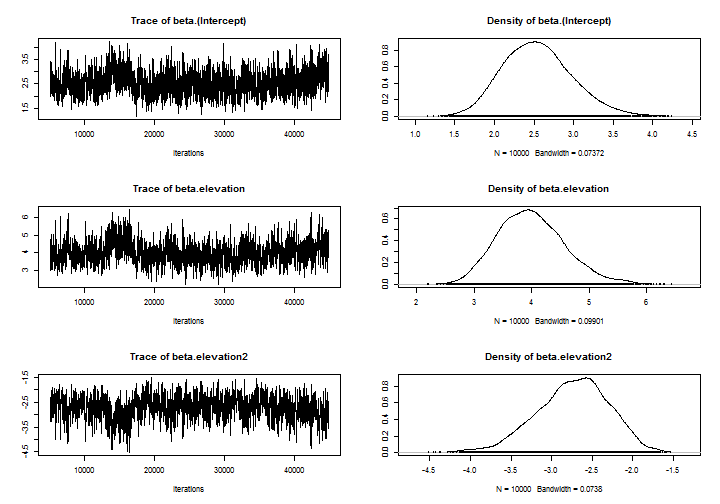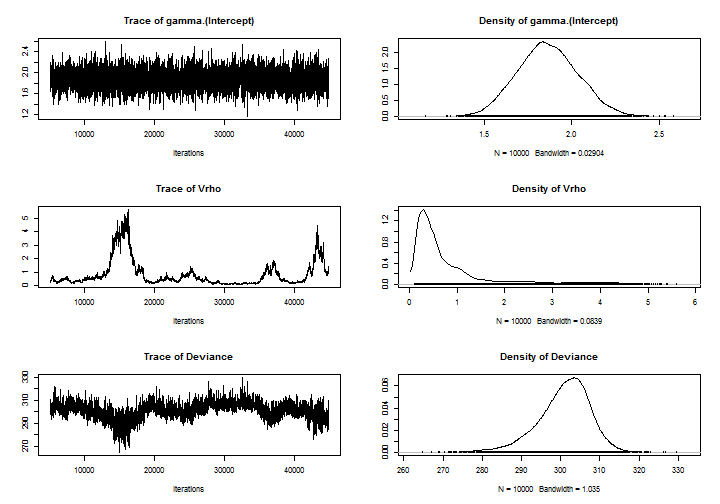# Extract the predictions and convert them to a raster object
rcar <- rasterFromXYZ(cbind(wbdata[, 1:2], newCAR$prob.p.pred)) # Extract the values of the spatial random effect and convert them to a # raster object rrho <- rasterFromXYZ(cbind(wbdata[, 1:2], newCAR$rho.pred))
plot(stack(rcar, rrho))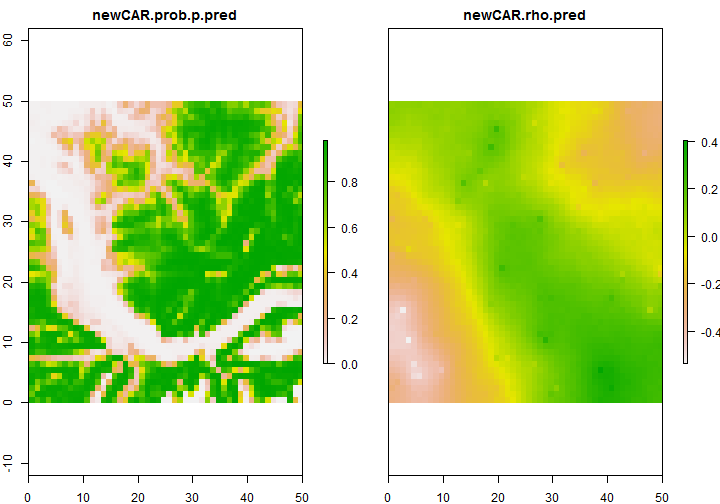We can compare our results with a naive model not accounting for imperfect detection and spatial autocorrelation. We define a site as occupied if we had at least one detection during the three visits, and we fit a standard logistic regression to predict the occupancy.

# Presence = detection for at least one visit
ynaive <- apply(yoccsample, 1, max)
ynaive <- ynaive[!is.na(ynaive)]

# Fit a logistic regression and compute the predictions for the whole study
# area
m <- glm(ynaive ~ elevation + I(elevation^2), family = binomial, data = sampledata)
predsnaive <- predict(m, newdata = fulldata, type = "response")

# Convert the predictions to a raster object
rnaive <- rasterFromXYZ(cbind(wbdata[, 1:2], predsnaive))
plot(stack(rcar, rnaive))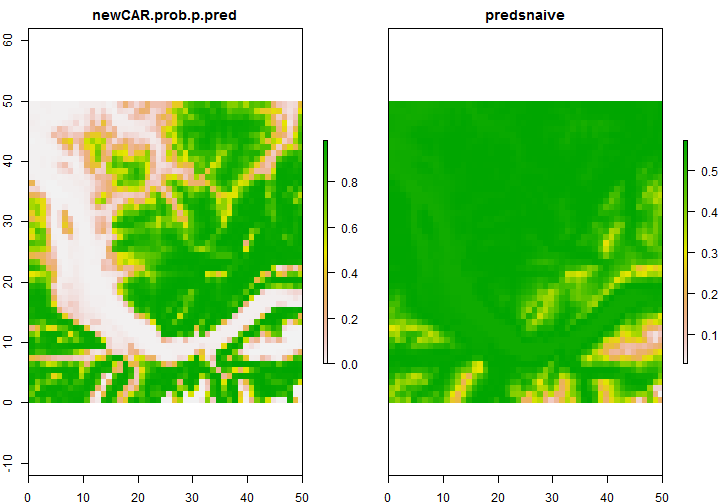As expected, we are getting much better results with the site-occupancy model accounting for spatial autocorrelation! Note that the hSDM package also allows modelling of counts accounting for imperfect detection and spatial autocorrelation, but it uses some obscure model instead of a more traditional N-mixture model.

### Analysis using the stocc package

The stocc package is relatively new and also allows us to model occupancy accounting for imperfect detection and spatial autocorrelation using a bayesian framework (Johnson et al., 2013). It is once again a site-occupancy with a CAR spatial random effect. The main difference with the hSDM package is that you can also use another type of (very recent) spatial model called RSR (restricted spatial regression). Indeed, one of the key limitations of the standard CAR model are the computing time for large study areas and a confounding potential between the covariate and the spatial random effect. This confounding may cause bias and will over inflate the uncertainty of the regression estimates. The stocc package is also using a probit link for occupancy instead of the standard logit link. This leads to more efficient MCMC sampling.

I don't claim to understand the RSR model, but here is very simplistic attempt to explain it. Basically the model will decompose a standard intrinsic CAR random effect into many independent components (the number of components is equal to the number of sites in your study area). When you fit the model, you only use a small proportion of these components (the authors recommend $$0.1*n$$). As a consequence the model fitting is faster and you have less confounding with the fixed effects.

Before going further, I'd like to acknowledge Kristin Broms for valuable help with the code of this chapter. As usual, we first need to format our data: the model needs two dataframes. The first one contains an ID for each site, the coordinates of the sites and the covariates used to model occupancy. The second dataframe contains the survey information: an ID for each site, the coordinate of each site, the covariates used to model detection probability and the detection/non-detection information. The surveys are not stored in a two-dimensional array but are stacked into one column. Note that the first dataframe will usually contain more sites than the second one: it will also contains the sites for which we want predictions. However the sites in the second dataframe have to be in the first dataframe, and the site IDs must of course correspond.

library(stocc)

## Loading required package: truncnorm

## Warning: package 'spam' was built under R version 3.0.2

## Loading required package: grid
## Spam version 0.40-0 (2013-09-11) is loaded.
## Type 'help( Spam)' or 'demo( spam)' for a short introduction
## and overview of this package.
## Help for individual functions is also obtained by adding the
## suffix '.spam' to the function name, e.g. 'help( chol.spam)'.
##
## Attaching package: 'spam'
##
## The following object is masked from 'package:base':
##
##     backsolve, forwardsolve
##
##
## This is stocc 1.0-7
## Built: R 3.0.1; ; 2013-09-03 16:06:24 UTC; windows
##
## Type "?stocc" for a description
##
## Type 'demo(simDataMCMC)' for a model fitting example.
## Be CAREFUL! The demo runs a full MCMC that can take a while
## The source for the demo can be obtained on your machine at 'C:/R/R-3.0.1/library/stocc/demo/simDataMCMC.R'


# Prepare the first dataframe
siteData <- data.frame(site = 1:n, x_coord = wbdata[, 1], y_coord = wbdata[,
2], elevation = fulldata$elevation, elevation2 = fulldata$elevation^2)

##   site x_coord y_coord elevation elevation2
## 1    1     0.5    49.5    -1.196      1.430
## 2    2     1.5    49.5    -1.177      1.385
## 3    3     2.5    49.5    -1.126      1.267
## 4    4     3.5    49.5    -1.135      1.288
## 5    5     4.5    49.5    -1.196      1.430
## 6    6     5.5    49.5    -1.247      1.555

# Prepare the second dataframe
surveyData <- data.frame(site = rep(sites, 3), x_coord = rep(wbdata[sites, 1],
3), y_coord = rep(wbdata[sites, 2], 3), PAData = as.vector(na.omit(yoccsample)))

##   site x_coord y_coord PAData
## 1 1506     5.5    19.5      1
## 2 1645    44.5    17.5      1
## 3 2255     4.5     4.5      0
## 4  304     3.5    43.5      0
## 5 1438    37.5    21.5      0
## 6 2267    16.5     4.5      1

# Group the surveys together
surveyData <- surveyData[order(surveyData$site), ] head(surveyData)  ## site x_coord y_coord PAData ## 134 88 37.5 48.5 1 ## 334 88 37.5 48.5 1 ## 534 88 37.5 48.5 0 ## 27 89 38.5 48.5 0 ## 227 89 38.5 48.5 0 ## 427 89 38.5 48.5 0  # Let the function know what is in your dataframes names <- list(visit = list(site = "site", obs = "PAData"), site = list(site = "site", coords = c("x_coord", "y_coord")))  We will first fit an intrinsic CAR model. As usual we model occupancy as a quadratic function of elevation and we assume a constant detection probability. The threshold argument is a distance used to define the neighbours (similar to the distance used in the dnearneigh function). Because we have a resolution of 1, all sites will have 8 neighbours (except those on the border). Because it is a bayesian analysis, we also need to specify a burnin, the number of iterations, the thinning rate, and some information for the priors. The initial values are automatically generated. We also fit an RSR model using the same function. The only difference is the content of the spatial.model argument. The moran.cut parameter controls the number of spatial components used in the model. Here we use 10% of the total number of sites. # Fit an ICAR model icar <- spatial.occupancy(detection.model = ~1, occupancy.model = ~elevation + elevation2, spatial.model = list(model = "icar", threshold = 1.5, rho = 1), so.data = make.so.data(surveyData, siteData, names), prior = list(a.tau = 0.05, b.tau = 0.005), control = list(burnin = 1000, iter = 10000, thin = 4))  ## ## Beginning MCMC routine ... ## ## Approximate time till completion: 0.71 hours ## ## 10 % completed ## ## 20 % completed ## ## 30 % completed ## ## 40 % completed ## ## 50 % completed ## ## 60 % completed ## ## 70 % completed ## ## 80 % completed ## ## 90 % completed ## ## 100 % completed   # Fit an RSR model rsr <- spatial.occupancy(detection.model = ~1, occupancy.model = ~elevation + elevation2, spatial.model = list(model = "rsr", threshold = 1.5, moran.cut = 0.1 * nrow(siteData)), so.data = make.so.data(surveyData, siteData, names), prior = list(a.tau = 0.01, b.tau = 0.01), control = list(burnin = 1000, iter = 10000, thin = 4))  ## ## Creating (R)estricted (S)patial (R)egression matrices ... ## ## Beginning MCMC routine ... ## ## Approximate time till completion: 0.52 hours ## ## 10 % completed ## ## 20 % completed ## ## 30 % completed ## ## 40 % completed ## ## 50 % completed ## ## 60 % completed ## ## 70 % completed ## ## 80 % completed ## ## 90 % completed ## ## 100 % completed  As usual we can plot the MCMC chains and the posterior densities. We can of course also extract the predictions and create a nice map for both models. # Trace and Density plots. plot(rsr$beta, main = "Detection Parameters")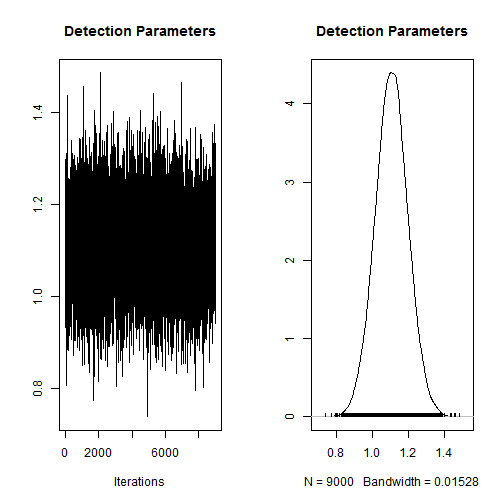plot(rsr$gamma, main = "Occupancy Parameters")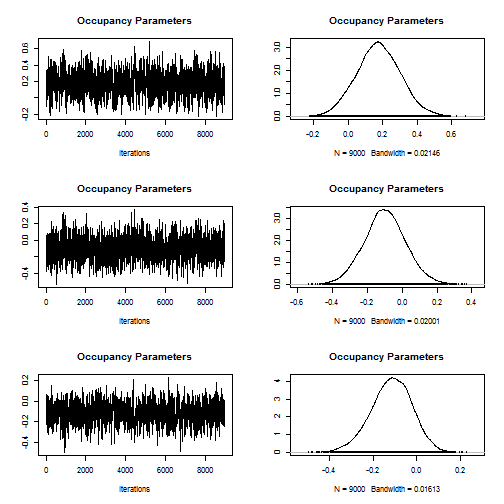plot(rsr$tau, main = "Variance Parameter")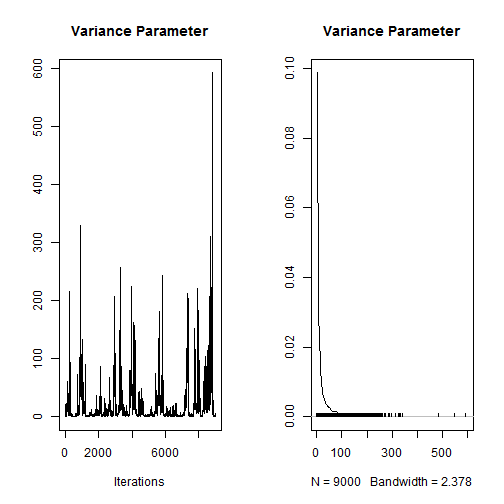rcar <- rasterFromXYZ(cbind(wbdata[, 1:2], icar$occupancy.df$psi.est))
plot(rcar)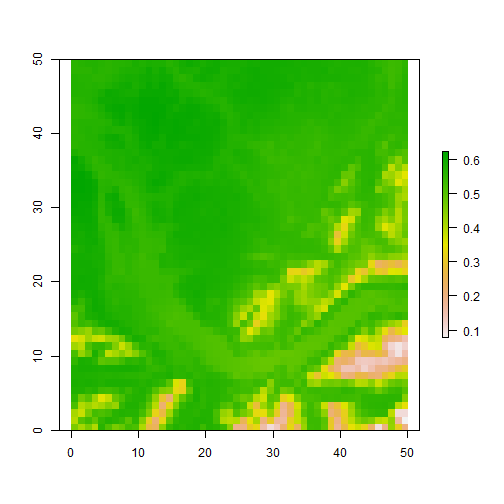rrsr <- rasterFromXYZ(cbind(wbdata[, 1:2], rsr$occupancy.df$psi.est))
plot(rrsr)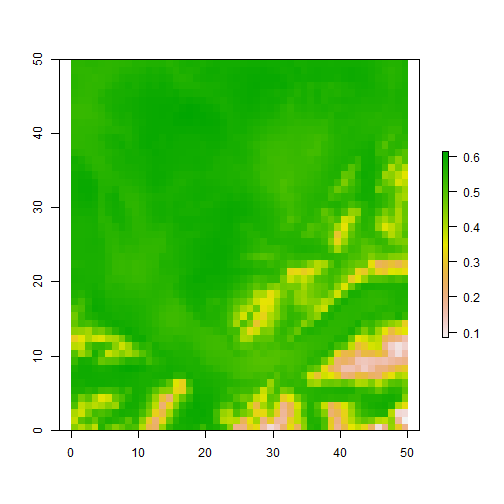We immediately see that the predictions are quite different compared to the ones we got with the hSDM package. They actually don't look so good. Actually the results of the intrinsic CAR model fitted with stocc should be similar to the results of hSDM. We don't really understand those differences but we note that if we increase the number of surveyed sites, the two packages give similar results (also the RSR model).

### Analysis using a dynamic autologistic model

The CAR models are a purely phenomenological way of accounting for spatial dependence. Often, we would want to specify more mechanistic models if we know how. In this section, we fit a dynamic occupancy model where a particular form of spatial dependence is explicitly modelled: due to dispersal from neighbouring quadrats, both survival and colonization probabilities are likely to be a positive function of local density around a focal quadrat (Bled et al., 2011).

We will illustrate this model class with data from the Swiss breeding bird survey MHB, specifically with data from the European crossbill. We are using data from 267 quadrats between 2000 and 2007, with 2-3 visits every year. The data preparation is a bit more complicated for this model (also because we are using a real dataset).

cb <- read.table("spatial.crossbill.txt", header = TRUE)
str(cb)

## 'data.frame':    267 obs. of  60 variables:
##  $id : int 1 2 3 4 5 6 7 8 9 10 ... ##$ x      : int  497500 503500 503500 509500 509500 521500 521500 527500 533500 533500 ...
##  $y : int 118500 134500 158500 150500 166500 150500 166500 174500 166500 182500 ... ##$ ele    : int  450 450 1050 950 1150 550 750 650 550 550 ...
##  $forest : int 3 21 32 9 35 2 6 60 5 13 ... ##$ surveys: int  3 3 3 3 3 3 3 3 3 3 ...
##  $det991 : int 0 0 NA 0 0 NA 0 0 0 0 ... ##$ det992 : int  0 0 NA 0 0 NA 0 0 0 0 ...
##  $det993 : int 0 0 NA 0 0 NA 0 0 0 0 ... ##$ det001 : int  0 0 0 1 1 0 0 0 0 1 ...
##  $det002 : int 0 0 0 0 1 0 0 0 0 0 ... ##$ det003 : int  0 0 0 0 1 0 1 0 0 0 ...
##  $det011 : int 0 0 1 0 0 0 NA 0 0 0 ... ##$ det012 : int  0 0 1 0 0 0 NA 0 0 0 ...
##  $det013 : int 0 0 0 0 1 0 NA 0 0 0 ... ##$ det021 : int  0 0 0 0 1 0 NA 0 0 0 ...
##  $det022 : int 0 0 0 0 0 0 NA 0 0 0 ... ##$ det023 : int  0 0 0 0 0 0 NA 0 0 1 ...
##  $det031 : int 0 0 1 0 1 0 0 0 NA 0 ... ##$ det032 : int  0 0 1 1 1 0 1 1 NA 0 ...
##  $det033 : int 0 0 1 0 0 0 0 0 NA 0 ... ##$ det041 : int  0 0 0 0 1 0 0 0 0 0 ...
##  $det042 : int 0 0 0 0 1 0 0 0 0 0 ... ##$ det043 : int  0 0 0 0 0 0 0 0 0 0 ...
##  $det051 : int 0 0 0 0 1 0 0 0 0 0 ... ##$ det052 : int  0 0 0 0 0 0 0 0 0 0 ...
##  $det053 : int 0 0 0 0 0 0 0 1 0 0 ... ##$ det061 : int  0 0 0 0 0 0 0 1 0 0 ...
##  $det062 : int 0 0 0 0 0 0 0 1 0 0 ... ##$ det063 : int  0 0 1 1 0 0 0 1 0 1 ...
##  $det071 : int 0 0 0 0 1 0 0 0 0 0 ... ##$ det072 : int  0 0 1 1 1 0 0 1 0 0 ...
##  $det073 : int 0 0 0 1 1 0 0 1 0 0 ... ##$ date991: int  34 17 NA 29 24 NA 26 23 21 37 ...
##  $date992: int 59 33 NA 59 45 NA 54 43 36 62 ... ##$ date993: int  65 65 NA 65 65 NA 74 71 56 75 ...
##  $date001: int 33 22 38 33 21 22 23 24 18 21 ... ##$ date002: int  48 50 67 48 46 35 37 37 31 49 ...
##  $date003: int 68 66 85 68 71 72 71 70 55 67 ... ##$ date011: int  30 14 28 24 31 27 NA 17 18 26 ...
##  $date012: int 54 28 50 54 56 55 NA 41 41 49 ... ##$ date013: int  76 61 83 76 73 80 NA 76 54 62 ...
##  $date021: int 29 13 30 39 23 28 NA 17 16 24 ... ##$ date022: int  58 39 47 61 44 56 NA 56 37 47 ...
##  $date023: int 73 62 74 77 71 73 NA 73 76 74 ... ##$ date031: int  24 18 26 31 16 24 37 31 NA 14 ...
##  $date032: int 49 40 55 62 44 51 60 45 NA 43 ... ##$ date033: int  76 68 87 76 70 60 74 71 NA 64 ...
##  $date041: int 28 23 25 24 22 27 20 21 34 20 ... ##$ date042: int  51 38 60 54 48 55 48 53 48 44 ...
##  $date043: int 74 59 95 74 69 64 55 75 67 70 ... ##$ date051: int  35 20 24 22 28 27 22 22 23 21 ...
##  $date052: int 52 35 59 60 51 39 43 41 49 36 ... ##$ date053: int  73 65 87 76 73 55 69 69 65 70 ...
##  $date061: int 19 15 23 22 23 29 29 24 23 21 ... ##$ date062: int  56 28 62 57 42 40 52 54 44 40 ...
##  $date063: int 65 55 90 78 70 52 65 69 66 68 ... ##$ date071: int  20 19 22 22 15 21 21 37 29 20 ...
##  $date072: int 46 50 55 49 43 40 53 50 43 40 ... ##$ date073: int  54 56 61 70 60 49 64 65 57 60 ...


# Extract response and survey dates and mean-impute dates
y.cross <- as.matrix(cb[, 10:33])  # survey data 2000-2007
DATE <- as.matrix(cb[, 37:60])  # Date of survey 2000-2007
sum(is.na(y.cross))  # Check how many NA's in response

##  426

sum(is.na(DATE))  # Check how many NA's in date covariate

##  457

cmeans.date <- apply(DATE, 2, mean, na.rm = TRUE)
for (j in 1:24) {
DATE[, j][is.na(DATE[, j])] <- cmeans.date[j]  # mean impute
}

# Then re-create same NA structure for response and date
DATE[is.na(y.cross)] <- NA
sum(is.na(y.cross))  # Check how many NA's in response: 426 both times

##  426

sum(is.na(DATE))  # Check how many NA's in date covariate

##  426


# Standardize covariates for elevation, forest, survey date
mean.ele <- mean(cb$ele, na.rm = TRUE) sd.ele <- sd(cb$ele, na.rm = TRUE)
elev <- (cb$ele - mean.ele)/sd.ele mean.forest <- mean(cb$forest, na.rm = TRUE)
sd.forest <- sd(cb$forest, na.rm = TRUE) forest <- (cb$forest - mean.forest)/sd.forest
mean.date <- mean(DATE, na.rm = TRUE)
sd.date <- sd(c(DATE), na.rm = TRUE)
DATE <- (DATE - mean.date)/sd.date

# Put survey data in 3D array and bundle all data
y <- array(NA, dim = c(267, 3, 8))  # sites by reps by years
for (k in 1:8) {
y[, , k] <- y.cross[, (3 * k - 2):(3 * k)]
}
str(y)

##  int [1:267, 1:3, 1:8] 0 0 0 1 1 0 0 0 0 1 ...


In order to perform the analysis we need to define a grid which will be the basis for defining the neighbourhood. We will use a grid with a 10 km resolution and treat multiple quadrats in the same cell as replicate observations. Once we have the grid, we can define our neighbours using the dnearneigh function. We will again use a second order neighbourhood (8 direct neighbours). For this model, we won't use the nb2WB function to convert our neighbourhood object, but we are going to define a matrix storing the neighbours for each site (see the adjmat matrix below).

# Create 10x10km grid across whole Switzerland
side.length <- 10000
coordkm <- cbind(ch$x, ch$y)
coordgrid <- unique(coordkm - (coordkm%%side.length) + 0.5 * side.length)

# Sort the grid cells and then create a cell ID...
coordgrid <- coordgrid[order(-coordgrid[, 2], coordgrid[, 1]), ]
spx <- cbind(coordgrid, 1:nrow(coordgrid))
# Check results
r <- rasterFromXYZ(spx)
plot(r, axes = FALSE, legend = FALSE)
text(r, cex = 0.5)

# Compute the neighborhood (2nd order)
neigh <- dnearneigh(coordgrid, d1 = 0, d2 = sqrt(2) * side.length + 1)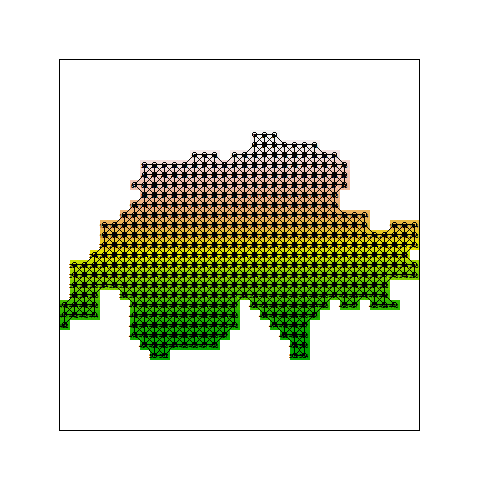# Store the neighborhood in the format needed by the BUGS model
cardneigh <- card(neigh)
adjmat <- matrix(nrow = length(neigh), ncol = max(cardneigh))
for (i in 1:length(neigh)) {
}

##      [,1] [,2] [,3] [,4] [,5] [,6] [,7] [,8]
## [1,]    2    4    5   NA   NA   NA   NA   NA
## [2,]    1    3    4    5    6   NA   NA   NA
## [3,]    2    5    6    7   NA   NA   NA   NA
## [4,]    1    2    5   15   16   17   NA   NA
## [5,]    1    2    3    4    6   16   17   18
## [6,]    2    3    5    7   17   18   19   NA


# Attribute the sites to the grid cells
gridmember <- extract(r, cbind(cb$x, cb$y))

# How many MHB quadrats per grid cell?
table(gridmember)

## gridmember
##   5   6  11  14  16  17  18  19  20  21  22  29  30  31  34  36  39  40
##   1   1   1   1   1   1   1   1   1   1   1   1   1   1   1   1   1   1
##  41  42  43  47  49  51  53  54  55  57  63  65  68  71  72  73  74  78
##   1   1   1   1   1   1   1   1   1   1   1   1   1   1   1   1   1   1
##  80  83  84  85  88  90  91  93  94  95  97  98 100 101 102 104 105 107
##   1   1   1   1   1   1   1   1   1   2   2   1   1   2   1   1   1   2
## 110 111 113 115 116 118 119 123 124 128 129 130 135 138 141 142 143 144
##   1   1   1   1   1   1   1   1   1   1   1   1   1   1   1   1   1   1
## 148 149 150 158 159 160 161 162 164 165 166 168 171 173 176 177 178 186
##   1   1   1   1   1   1   1   1   1   1   1   1   1   1   1   1   1   2
## 189 190 191 194 195 196 197 198 199 200 202 203 204 205 206 207 209 210
##   1   1   1   1   2   1   1   1   1   1   2   1   1   1   1   1   1   2
## 211 214 215 218 219 220 221 224 226 229 231 232 237 238 239 241 243 244
##   1   1   1   1   1   1   1   1   1   2   1   1   1   2   1   2   1   1
## 245 250 253 255 256 257 259 260 262 263 268 269 271 272 282 284 285 287
##   1   1   1   1   1   1   1   1   1   1   1   1   1   1   1   1   1   1
## 288 292 293 296 300 302 304 306 307 309 311 314 317 319 320 321 322 324
##   1   1   1   1   1   1   1   1   1   2   1   1   2   1   1   1   1   1
## 325 326 328 329 330 333 335 336 337 338 341 342 343 346 358 359 360 361
##   1   2   1   1   1   1   2   3   2   1   1   1   2   1   1   1   1   1
## 363 364 367 373 375 379 381 384 387 390 393 394 396 397 400 401 402 404
##   1   1   1   1   1   2   1   1   1   1   1   1   1   1   1   1   1   1
## 405 408 412 418 420 421 422 424 425 426 428 429 430 431 432 434 439 442
##   1   1   1   1   1   1   1   1   1   1   1   1   1   1   1   1   1   1
## 445 446 447 448 449 451 452 454 456 457 458 459 466 468 470 476 480 481
##   1   1   1   2   1   2   1   3   1   1   1   2   1   1   1   3   1   1
## 484 485 489 493 500 503
##   1   1   1   1   1   1

table(table(gridmember))

##
##   1   2   3
## 216  21   3


# Produce map of the grid
plot(rasterToPolygons(r))
points(cb[, c("x", "y")], col = "blue", pch = 16, cex = 0.5)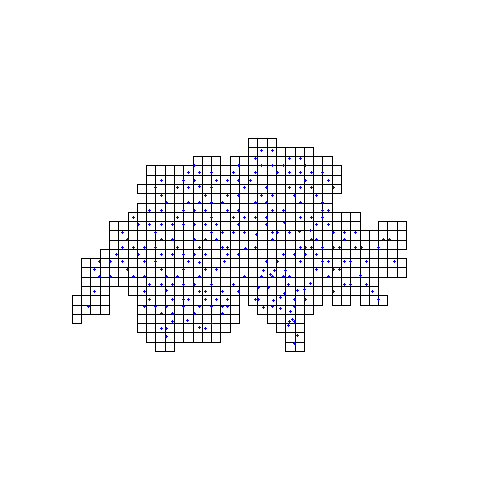We can now write the model in the BUGS language. This is actually a dynamic site-occupancy with a special covariate used to model the colonisation and the persistence probabilities. This covariate describes, for each site, the proportion of occupied neighbouring cells. We don't use the observed state to compute this proportion (because there's imperfect detection), but we use the latent occurence state (the $$z_i$$ values).

# Specify model in BUGS language
sink("Dynocc.txt")
cat("
model {

# Specify priors
psi1 ~ dunif(0, 1)
for (k in 1:(nyear-1)){
alpha.lphi[k] ~ dnorm(0, 0.01)
alpha.lgamma[k] ~ dnorm(0, 0.01)
logit(p[k]) <- lp[k]
lp[k] ~ dnorm(0, 0.01)
}
logit(p[nyear]) <- lp[nyear]
lp[nyear] ~ dnorm(0, 0.01)
beta.lphi ~ dnorm(0, 0.01)
beta.lgamma ~ dnorm(0, 0.01)

# Ecological submodel: Define latent states of occurrence for each cell
for (i in 1:ncell){
z[i,1] ~ dbern(psi1)
for (k in 2:nyear){

# Compute local density (prop of occupied neighbouring cells) for
# use as autologistic covariate
for (m in 1:cardneigh[i]){
# Estimated (latent) occurrence state of all neighbours
}
z[i,k] ~ dbern(muZ[i,k])
muZ[i,k]<- z[i,k-1]*phi[i,k-1] + (1-z[i,k-1])*gamma[i,k-1]

# Proportion of neighbours occupied (auto-covariate)
D[i,k-1] <- sum(ConnSite[i, 1:cardneigh[i], k-1]) / cardneigh[i]
logit(gamma[i, k-1]) <- alpha.lgamma[k-1] + beta.lgamma * D[i, k-1]
logit(phi[i, k-1]) <- alpha.lphi[k-1] + beta.lphi * D[i, k-1]
}
}

# Observation model
for (i in 1:nsite){
for (j in 1:nrep){
for (k in 1:nyear){
muy[i,j,k] <- z[gridmember[i],k]*p[k]
y[i,j,k] ~ dbern(muy[i,j,k])
}
}
}
}
", fill = TRUE)
sink()


We now have to bundle the data, to define some initial values and the MCMC settings.

# Bundle data
win.data <- list(y = y, nsite = dim(y), nrep = dim(y), nyear = dim(y),

# Initial values
inits <- function() {
list(z = zst)
}

# Parameters monitored
params <- c("psi1", "alpha.lphi", "beta.lphi", "alpha.lgamma", "beta.lgamma",
"p", "z")

# MCMC settings
ni <- 2500
nt <- 2
nb <- 500
nc <- 3


And we can now run the model in JAGS (or in WinBUGS). This is a complex model and therefore it takes quite a long time to run it. Moreover, convergence can be difficult to achieve…

library(R2jags)

## Loading required package: rjags
##
## Attaching package: 'R2jags'
##
## The following object is masked from 'package:coda':
##
##     traceplot

# Call JAGS from R
outJAGS <- jags(win.data, inits, params, "Dynocc.txt", n.chains = nc, n.thin = nt,
n.iter = ni, n.burnin = nb)

## module glm loaded

## Compiling model graph
##    Resolving undeclared variables
##    Allocating nodes
##    Graph Size: 62697
##
## Initializing model


We can now produce dynamic distribution maps for the crossbill in Switzerland between 2000-2007. Note that, in this example, we are plotting one possible realisation of the latent occurrrence state and not the occupancy probability (but this is of course possible).

# traceplot(outJAGS)
print(outJAGS$BUGSoutput$summary[1:40, ], dig = 3)

##                      mean      sd     2.5%      25%      50%      75%
## alpha.lgamma   -3.2255  0.5828   -4.427   -3.585   -3.193   -2.814
## alpha.lgamma   -1.7722  0.4431   -2.744   -2.050   -1.745   -1.468
## alpha.lgamma   -2.6487  0.6031   -3.868   -3.027   -2.641   -2.242
## alpha.lgamma   -6.4211  4.5136  -19.474   -6.709   -4.573   -3.806
## alpha.lgamma   -3.7336  0.7035   -5.184   -4.116   -3.666   -3.271
## alpha.lgamma   -2.9720  0.6350   -4.242   -3.383   -2.942   -2.552
## alpha.lgamma   -4.5836  2.6354  -13.254   -4.600   -3.802   -3.249
## alpha.lphi     -0.5209  0.7532   -1.927   -0.992   -0.567   -0.101
## alpha.lphi      1.9641  2.1985    0.154    0.965    1.465    2.098
## alpha.lphi      1.0836  0.7658   -0.263    0.621    1.027    1.496
## alpha.lphi      0.7756  0.7840   -0.627    0.289    0.737    1.187
## alpha.lphi      0.9743  0.8830   -0.632    0.413    0.922    1.433
## alpha.lphi      0.8740  0.8311   -0.576    0.375    0.835    1.283
## alpha.lphi      0.8953  0.9039   -0.491    0.326    0.795    1.316
## beta.lgamma        2.5295  0.8566    0.794    1.934    2.591    3.145
## beta.lphi          2.0179  1.3742   -1.270    1.327    2.050    2.798
## deviance        3682.7747 60.3400 3564.757 3640.578 3681.332 3723.411
## p               0.5634  0.0336    0.497    0.541    0.564    0.586
## p               0.4542  0.0487    0.355    0.421    0.455    0.488
## p               0.4080  0.0353    0.339    0.384    0.407    0.432
## p               0.4970  0.0304    0.438    0.477    0.497    0.517
## p               0.4326  0.0332    0.370    0.409    0.431    0.455
## p               0.5558  0.0346    0.489    0.532    0.555    0.580
## p               0.3152  0.0315    0.259    0.293    0.314    0.336
## p               0.5339  0.0340    0.465    0.511    0.534    0.557
## psi1               0.4083  0.0356    0.340    0.384    0.408    0.432
## z[1,1]             0.4510  0.4977    0.000    0.000    0.000    1.000
## z[2,1]             0.4737  0.4994    0.000    0.000    0.000    1.000
## z[3,1]             0.4483  0.4974    0.000    0.000    0.000    1.000
## z[4,1]             0.4880  0.4999    0.000    0.000    0.000    1.000
## z[5,1]             1.0000  0.0000    1.000    1.000    1.000    1.000
## z[6,1]             0.0480  0.2138    0.000    0.000    0.000    0.000
## z[7,1]             0.4203  0.4937    0.000    0.000    0.000    1.000
## z[8,1]             0.3627  0.4808    0.000    0.000    0.000    1.000
## z[9,1]             0.3610  0.4804    0.000    0.000    0.000    1.000
## z[10,1]            0.3587  0.4797    0.000    0.000    0.000    1.000
## z[11,1]            0.0393  0.1944    0.000    0.000    0.000    0.000
## z[12,1]            0.4343  0.4958    0.000    0.000    0.000    1.000
## z[13,1]            0.3713  0.4832    0.000    0.000    0.000    1.000
## z[14,1]            0.0237  0.1520    0.000    0.000    0.000    0.000
##                    97.5% Rhat n.eff
## alpha.lgamma   -2.197 1.03    70
## alpha.lgamma   -0.976 1.06    53
## alpha.lgamma   -1.509 1.02    93
## alpha.lgamma   -2.848 1.01   720
## alpha.lgamma   -2.561 1.02   980
## alpha.lgamma   -1.785 1.01   240
## alpha.lgamma   -2.469 1.02   800
## alpha.lphi      1.123 1.11    28
## alpha.lphi      9.731 1.13    95
## alpha.lphi      2.812 1.07    42
## alpha.lphi      2.454 1.08    36
## alpha.lphi      3.239 1.05    47
## alpha.lphi      2.701 1.08    34
## alpha.lphi      2.976 1.10    35
## beta.lgamma        4.011 1.04    74
## beta.lphi          4.728 1.14    23
## deviance        3804.172 1.01   230
## p               0.630 1.00  3000
## p               0.546 1.01   290
## p               0.477 1.00   690
## p               0.557 1.00   600
## p               0.502 1.01   190
## p               0.622 1.00   730
## p               0.381 1.00  2400
## p               0.599 1.00  3000
## psi1               0.479 1.00  2400
## z[1,1]             1.000 1.00   800
## z[2,1]             1.000 1.00  1600
## z[3,1]             1.000 1.00  1400
## z[4,1]             1.000 1.00  1500
## z[5,1]             1.000 1.00     1
## z[6,1]             1.000 1.00  2900
## z[7,1]             1.000 1.00  3000
## z[8,1]             1.000 1.00   860
## z[9,1]             1.000 1.00  2100
## z[10,1]            1.000 1.00  3000
## z[11,1]            1.000 1.00  3000
## z[12,1]            1.000 1.00  1000
## z[13,1]            1.000 1.00  3000
## z[14,1]            0.000 1.01  2700

# Store the results in a SpatialPixelsDataFrame
spres <- data.frame(cbind(spx, outJAGS$BUGSoutput$mean\$z[, 1:8]))
coordinates(spres) <- spres[, 1:2]
gridded(spres) <- TRUE
# Convert to raster object and plot
r <- brick(spres[4:11])
names(r) <- paste("Distribution map", 2000:2007)
plot(r, axes = FALSE)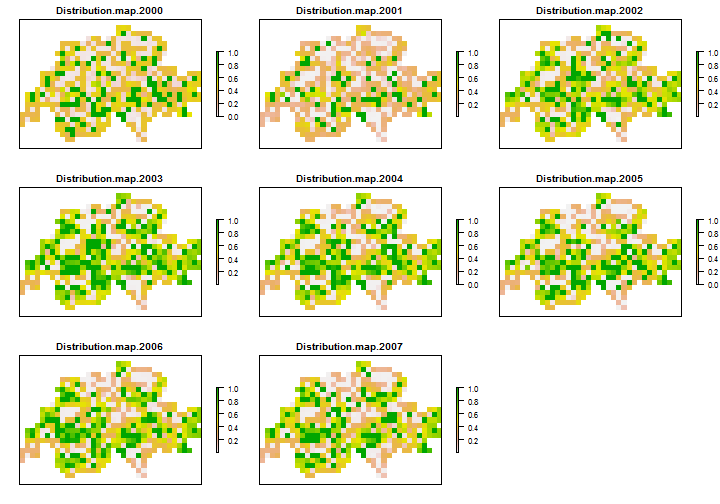Obvious extensions would be the inclusion of environmental covariates (e.g. elevation and forest cover or proportion of coniferous trees) or additional spatial components, such as CAR random effects on initial occupancy and detection. The latter may be needed to account for spatially correlated abundance, which is manifest as spatially correlated detection (R. Altwegg, personal communication).